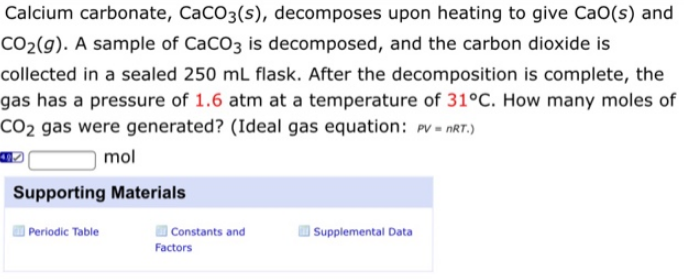# Problem: Calcium carbonate, CaCO3(s), decomposes upon heating to give CaO(s) and CO2(g). A sample of CaCO3 is decomposed, and the carbon dioxide is collected in a sealed 250 mL flask. After the decomposition is complete, the gas has a pressure of 1.6 atm at a temperature of 31 °C. How many moles of CO2 gas were generated? (Ideal gas equation: PV = nRT.)

###### FREE Expert Solution
84% (89 ratings)###### Problem Details

Calcium carbonate, CaCO3(s), decomposes upon heating to give CaO(s) and CO2(g). A sample of CaCO3 is decomposed, and the carbon dioxide is collected in a sealed 250 mL flask. After the decomposition is complete, the gas has a pressure of 1.6 atm at a temperature of 31 °C. How many moles of CO2 gas were generated? (Ideal gas equation: PV = nRT.)